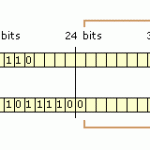Recently I was working to track down a multicast problem for a client on an enterprise network and needed to determine what the exact multicast MAC address would be. I knew that any mac address that started with 01-00-5E was a multicast address, but wasn’t sure how the rest of it was calculated. I’ll explain below exactly how to determine the multicast MAC address from the multicast IP as well as provide a few helpful websites.

Overview

At the layer 2 level whenever multicast is used it has to be assigned a MAC address in order to work. There is a specific range assigned for multicast, 01-00-5E-00-00-00 to 01-00-5E-7F-FF-FF for Ethernet and Fiber Distributed Data Interface (FDDI). As I explained above, any MAC address you see that starts with 01-00-5E is related to a multicast address. A MAC address is made up of 48-bits. The high order 25 bits of the MAC address are fixed and that is the 01-00-5E. To determine the rest of the MAC address we will use the remaining 23 low order bits to generate the full MAC address. You should have a good understanding of IP sub-netting or this may get a bit confusing.Example Used Throughout Tutorial

Multicast IP: 239.64.100.1

Convert Second Octet of Multicast IP to Binary

The first step is to convert the second octet of the multicast IP to binary. In the example I will be using the second octet of the multicast IP 239.64.100.1 which would be 64. A quick way to do this is by following this example below. Start from left to right. Can 128 be subtracted from 64? No, write a 0. Can 64 be subtracted from 64? Yes, write a 1. You keep doing this until you can no longer subtract from the octet and you have converted decimal to binary. Easy right?

128 64 32 16 8 4 2 1
0    1    0   0 0 0 0 0

So above we converted the second octet of our multicast IP which was 64 to decimal which equal 01000000.

64 decimal = 01000000 binary

Since we are only using the last 7 bits from the second octet, just drop the first 0 or 1 from our binary number (first binary number on the left, the result from 128 above).

Last 7 bits of 64 which still equals 64 converted to binary = 1000000

After removing 1 bit from our binary result it still equals 64 so this is what we will be using in the next step.

I could have explained this above but then you would have skipped through this part which does make a difference. Had our multicast IP been 239.192.100.1, that would mean our binary would be 11000000. After dropping that first bit it changes the binary to 64 instead of 192! Do not skip this part.

Now that we have determined what the last seven bits of our second octet are we can convert it to hexadecimal. Hexadecimal numbers use a base 16 where numbers are counted from 0 to 9 then letters A to F before adding another digit. You can think of it as though A is 10, B is 11, C is 12, D is 13, E is 14, and F is 15. Using this method makes it much easier to work with large numbers over using decimal standard. Decimals use a base 10 while Hexadecimals use a base 16.

You can use this website to automatically convert it for you:
http://www.binaryhexconverter.com/decimal-to-hex-converter

So from our example, converting 64 to hexadecimal equals 40 or (0x40).

Now we use this for the next part of our MAC Address. Using the first 01-00-5E, we now add 40, which makes the MAC 01-00-5E-40.

Next we will take the two last octets and convert those to hexadecimal.

100 becomes 64 in decimal, so add that to the MAC and it becomes 01-00-5E-40-64

1 becomes 1, add that to the MAC and we get our full MAC address 01-00-5E-40-64-01

So, multicast IP of 239.64.100.1 will have a MAC address of 01-00-5E-40-64-01

I’ll finish up the ending later and add instructions to convert decimal to hex.

This is still a work in progress.

http://www.computerhope.com/binhex.htmOrlando, FL